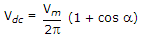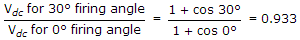# Electronics and Communication Engineering - Power Electronics - Discussion

### Discussion :: Power Electronics - Section 1 (Q.No.50)

50.

A single phase half wave rectifier is feeding a resistive load. When a = 0, dc output voltage is 127 V. When a = 30°, the dc output voltage will be about

 [A]. 127 V [B]. 120 V [C]. 110 V [D]. 100 V

Explanation:

Using Eq= 0.933.

Hence Vdc for 30° firing angle = 0.933 x 127 = ≈ 120 V.